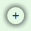# Profit Analysis

As opposite to break-even, companies are more interested in realizing profits. Profit Analysis, is a mathematical computation that helps a business identify the point where it reaches a specific target of profit.

This profit analysis calculator allows a business to accomplish the following:

• Determine the quantity it needs to produce or sell in order to realize a specific profit;
• Determine the selling price it needs to charge for a specific quantity you sell in order to realize a specific profit.
 Input Results Selling Price per Unit: Target units: 0.00 Fixed Cost: Total Revenue: \$ 0.00 Variable Cost per Unit: Total Cost: \$ 0.00 Target Profit: Profit: \$ 0.00Change variables Show details Note: This calculator is a JavaScript program. You must have JavaScript enabled in order to use it.

## Definitions and terms used in the Profit Analysis

• Selling Price per Unit: the price that a unit is expected to be sold for.
• Selling Units: the number of units expected to be sold (determined by a contract or market research).
• Fixed Cost (FC): the cost that remains constant within a range of production or sales, regardless of the number of units produced or sold within that range. Typical fixed costs are: rent, mortgage, equipment, salaries, insurance, fixed utilities (office utilities) etc.
• Variable Cost per Unit: the cost that vary with the production or the purchase of one unit.
• Target Profit: the profit that the company intend to realize.
• Target point: the point where the Target Profit is realized.
• Total Variable Cost (VC): the cost that varies directly with the number of units produced or sold. Typical variable costs are: materials, packaging and shipping, sales commission, hourly wages, variable utilities (factory utilities) etc.

Total Variable Cost = Selling Units x Variable Cost per Unit
• Total Cost (TC): total expenses incurred in the process of producing or selling a number of units.

Total Cost (TC) = Fixed Cost (FC) + Total Variable Cost (VC)
• Total Revenue: the total sales value of the units produced or sold.

Total Revenue = Selling Units x Selling Price per Unit
• Profit: the benefits from producing or selling a number of units.

Profit = Total Revenue - Total Cost
• Profit margin: the ratio of profitability calculated as Profit divided by Total Revenue. Profit margin is an indicator of a company's pricing policies and its ability to control costs. It measures how much out of every dollar of sales a company actually keeps in earnings (expressed as a percentage).

Profit Margin = (Total Revenues - Total Cost) / Total Revenues x 100
• Markup: the amount added to the cost of a product to cover expenses and profit in fixing the selling price. Markup is equal to profit.
• Markup percent: the ratio calculated as Markup divided by Total Cost. It measures how much is added to the cost in order to determine the selling price.

Markup percent = (Total Revenues - Total Cost) / Total Cost x 100